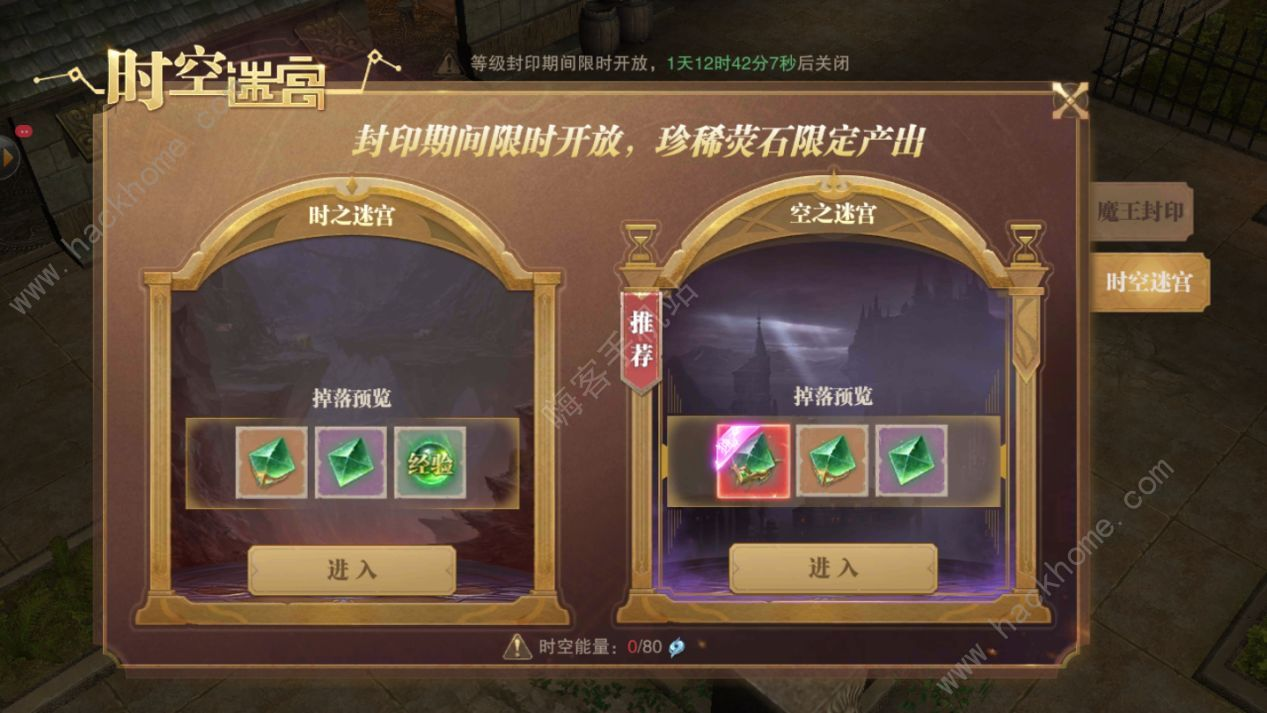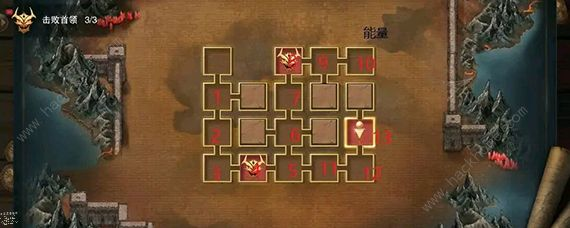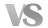110+90+90-210=80

80+90+30-60=140

140+30-130=40

40+90-40=90

90+60-150＝00+90

90+30-60=60

60+30-90=0

90+60=150-150=0

50%

050%

0

(您的评论需要经过审核才能显示)